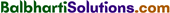By going through these Maharashtra State Board 12th Science Chemistry Notes Chapter 5 Electrochemistry students can recall all the concepts quickly.

## Maharashtra State Board 12th Chemistry Notes Chapter 5 Electrochemistry

→ Electrical conductance (G) = $$\frac{1}{R}$$Ω-1 2 or S

→ Cell constant = $$\frac{l}{a}$$ cm-1 (m-1)

→ Conductivity(k) = $$\frac{\text { Cell constant }}{\text { Resistance }}$$

→ Molar Conductivity(∧m) = $$\frac{\kappa}{C}$$ (k in Ω-1 m-1 and C in mol-3) OR
m = $$\frac{\kappa \times 1000}{C}$$ (k in Ω-1 cm-1 and C in mol dm-3)

→ Kohlrausch’s law : ∧0 = $$\lambda_{+}^{0}+\lambda_{-}^{0}$$

→ Degree of dissociation (α) = $$\frac{\Lambda_{\mathrm{m}}}{\Lambda_{0}}$$→ Dissociation constant (Ka) = $$\frac{\bigwedge_{\mathrm{m}}^{2} C}{\Lambda_{0}\left(\Lambda_{0}-\Lambda_{\mathrm{m}}\right)}$$

→ 1 Faraday = 96500 C (Change on one mole of electrons)

→ E°cell = E°red (cathode) – E°red (anode)

→ EMn+/M = E°Mn+/M – $$\frac{0.0592}{n}$$ log10[Mn+]

→ Ecell = E°cell – $$\frac{0.0592}{n} \log _{10} \frac{[\text { Products }]}{[\text { Reactants }]}$$

→ ΔG° = – nFE°cell

→ ΔG = -nFEcell

→ ΔG°= -RTlnK

→ E°cell = $$\frac{0.0592}{n}$$ log10K
For spontaneous cell reaction : Ecell > 0; ΔG < 0